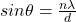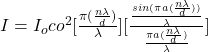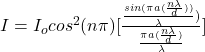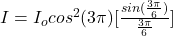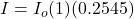## (a) How many fringes appear between the first diffraction-envelope minima to either side of the central maximum in a double-slit pattern if

Question

(a) How many fringes appear between the first diffraction-envelope minima to either side of the central maximum in a double-slit pattern if λ = 471 nm, d = 0.117 mm, and a = 35.7 µm? (b) What is the ratio of the intensity of the third bright fringe to the intensity of the central fringe?

in progress 0
6 months 2021-07-29T20:19:32+00:00 1 Answers 10 views 0

a

The number of fringe is  z  = 3 fringes

b

The  ratio is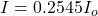Explanation:

a

From the question we are told that

The wavelength is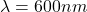The distance between the slit is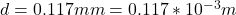The width of the slit is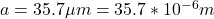let  z be the number of fringes that appear between the first diffraction-envelope minima to either side of the central maximum in a double-slit pattern is  and this mathematically represented as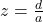Substituting values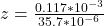z  = 3 fringes

b

From the question  we are told that the order  of the bright fringe is  n = 3

Generally the intensity of  a pattern  is mathematically represented as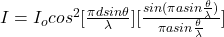Where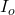is the intensity  of the  central fringe

And  Generally